# The angle of view

Determine the angle of view at which the observer sees a rod 16 m long when it is 18 m from one end and 27 m from the other.

Result

A =  34.919 °

#### Solution:

$a=16 \ \text{m} \ \\ b=18 \ \text{m} \ \\ c=27 \ \text{m} \ \\ \ \\ a^2=b^2+c^2 - 2 \cdot \ b \cdot \ c \cdot \ \cos A \ \\ \ \\ x=\dfrac{ -a^2+b^2+c^2 }{ 2 \cdot \ b \cdot \ c }=\dfrac{ -16^2+18^2+27^2 }{ 2 \cdot \ 18 \cdot \ 27 } \doteq \dfrac{ 797 }{ 972 } \doteq 0.82 \ \\ \ \\ A=\dfrac{ 180^\circ }{ \pi } \cdot \arccos(x)=\dfrac{ 180^\circ }{ \pi } \cdot \arccos(0.82) \doteq 34.9193 \doteq 34.919 ^\circ \doteq 34^\circ 55'10"$

Try calculation via our triangle calculator.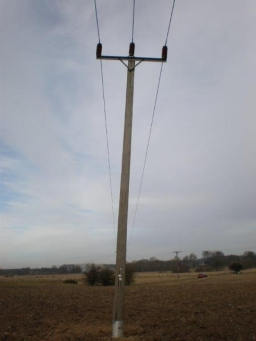Our examples were largely sent or created by pupils and students themselves. Therefore, we would be pleased if you could send us any errors you found, spelling mistakes, or rephasing the example. Thank you!

Leave us a comment of this math problem and its solution (i.e. if it is still somewhat unclear...):Be the first to comment!Tips to related online calculators
Cosine rule uses trigonometric SAS triangle calculator.

## Next similar math problems:

1. Tetrahedral pyramidDetermine the surface of a regular tetrahedral pyramid when its volume is V = 120 and the angle of the sidewall with the base plane is α = 42° 30´.
2. Isosceles triangle 10In an isosceles triangle, the equal sides are 2/3 of the length of the base. Determine the measure of the base angles.The angle of a straight road is approximately 12 degrees. Determine the percentage of this road.
4. Power line poleFrom point A, the power line pole is seen at an angle of 18 degrees. From point B to which we get when going from point A 30m away from the column at an angle of 10 degrees. Find the height of the power pole.
5. Church tower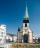Archdeacon church in Usti nad Labem has diverted tower by 186 cm. The tower is 65 m high. Calculate the angle by which the tower is tilted. Result write in degree's minutes.
6. Ratio iso triangleThe ratio of the sides of an isosceles triangle is 7:6:7 Find the base angle to the nearest answer correct to 3 significant figure.
7. The rescue helicopterThe rescue helicopter is above the landing site at a height of 180m. The site of the rescue operation can be seen from here at a depth angle of 52° 40 '. How far will the helicopter land from the rescue site?
8. What percentageWhat percentage of the Earth’s surface is seen by an astronaut from a height of h = 350 km. Take the Earth as a sphere with the radius R = 6370 km
9. The aspect ratioThe aspect ratio of the rectangular triangle is 13: 12: 5. Calculate the internal angles of the triangle.
10. Diagonals of pentagonCalculate the diagonal length of the regular pentagon: a) inscribed in a circle of radius 12dm; b) a circumscribed circle with a radius of 12dm.
11. Trapezoid MOThe rectangular trapezoid ABCD with right angle at point B, |AC| = 12, |CD| = 8, diagonals are perpendicular to each other. Calculate the perimeter and area of ​​the trapezoid.
12. Two forcesTwo forces with magnitudes of 25 and 30 pounds act on an object at angles of 10° and 100° respectively. Find the direction and magnitude of the resultant force. Round to two decimal places in all intermediate steps and in your final answer.
13. Steps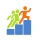Find the height between the two floors if you know that the number of steps between the two floors is 18, the gradient is 30º and the length of the step is 28.6 cm. Report the result in centimeters to the nearest centimeter.
14. Right triangleCalculate the length of the remaining two sides and the angles in the rectangular triangle ABC if a = 10 cm, angle alpha = 18°40'.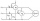Two wattmeters are connected to measuring power in a 3 phase balanced load. Determine the total power and power factor if the two wattmeters read 1000 watts each (1) both positive and (2) second reading is negative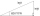One side is 36 long with a 15° incline. What is the height at the end of that side?I have coordinates of square vertices A / -3; 1/and B/1; 4 /. Find coordinates of vertices C and D, C 'and D'. Thanks Peter.